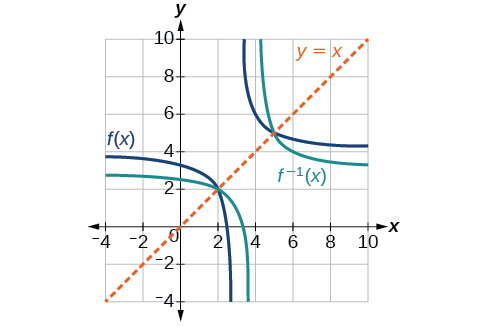3.7 Inverse functions  (Page 6/9)

 Page 6 / 9

Draw graphs of the functions and from [link] .Is there any function that is equal to its own inverse?

Yes. If $\text{\hspace{0.17em}}f={f}^{-1},\text{\hspace{0.17em}}$ then $\text{\hspace{0.17em}}f\left(f\left(x\right)\right)=x,\text{\hspace{0.17em}}$ and we can think of several functions that have this property. The identity function does, and so does the reciprocal function, because

$\frac{1}{\frac{1}{x}}=x$

Any function $\text{\hspace{0.17em}}f\left(x\right)=c-x,\text{\hspace{0.17em}}$ where $\text{\hspace{0.17em}}c\text{\hspace{0.17em}}$ is a constant, is also equal to its own inverse.

Access these online resources for additional instruction and practice with inverse functions.

Visit this website for additional practice questions from Learningpod.

Key concepts

• If $\text{\hspace{0.17em}}g\left(x\right)\text{\hspace{0.17em}}$ is the inverse of $\text{\hspace{0.17em}}f\left(x\right),\text{\hspace{0.17em}}$ then $\text{\hspace{0.17em}}g\left(f\left(x\right)\right)=f\left(g\left(x\right)\right)=x.\text{\hspace{0.17em}}$ See [link] , [link] , and [link] .
• Only some of the toolkit functions have an inverse. See [link] .
• For a function to have an inverse, it must be one-to-one (pass the horizontal line test).
• A function that is not one-to-one over its entire domain may be one-to-one on part of its domain.
• For a tabular function, exchange the input and output rows to obtain the inverse. See [link] .
• The inverse of a function can be determined at specific points on its graph. See [link] .
• To find the inverse of a formula, solve the equation $\text{\hspace{0.17em}}y=f\left(x\right)\text{\hspace{0.17em}}$ for $\text{\hspace{0.17em}}x\text{\hspace{0.17em}}$ as a function of $\text{\hspace{0.17em}}y.\text{\hspace{0.17em}}$ Then exchange the labels $\text{\hspace{0.17em}}x\text{\hspace{0.17em}}$ and $\text{\hspace{0.17em}}\text{\hspace{0.17em}}y.\text{\hspace{0.17em}}\text{\hspace{0.17em}}$ See [link] , [link] , and [link] .
• The graph of an inverse function is the reflection of the graph of the original function across the line $\text{\hspace{0.17em}}y=x.\text{\hspace{0.17em}}$ See [link] .

Verbal

Describe why the horizontal line test is an effective way to determine whether a function is one-to-one?

Each output of a function must have exactly one output for the function to be one-to-one. If any horizontal line crosses the graph of a function more than once, that means that $\text{\hspace{0.17em}}y$ -values repeat and the function is not one-to-one. If no horizontal line crosses the graph of the function more than once, then no $\text{\hspace{0.17em}}y$ -values repeat and the function is one-to-one.

Why do we restrict the domain of the function $\text{\hspace{0.17em}}f\left(x\right)={x}^{2}\text{\hspace{0.17em}}$ to find the function’s inverse?

Can a function be its own inverse? Explain.

Yes. For example, $\text{\hspace{0.17em}}f\left(x\right)=\frac{1}{x}\text{\hspace{0.17em}}$ is its own inverse.

Are one-to-one functions either always increasing or always decreasing? Why or why not?

How do you find the inverse of a function algebraically?

Given a function $\text{\hspace{0.17em}}y=f\left(x\right),\text{\hspace{0.17em}}$ solve for $\text{\hspace{0.17em}}x\text{\hspace{0.17em}}$ in terms of $\text{\hspace{0.17em}}y.\text{\hspace{0.17em}}$ Interchange the $\text{\hspace{0.17em}}x\text{\hspace{0.17em}}$ and $\text{\hspace{0.17em}}y.\text{\hspace{0.17em}}$ Solve the new equation for $\text{\hspace{0.17em}}y.\text{\hspace{0.17em}}$ The expression for $\text{\hspace{0.17em}}y\text{\hspace{0.17em}}$ is the inverse, $\text{\hspace{0.17em}}y={f}^{-1}\left(x\right).$

Algebraic

Show that the function $\text{\hspace{0.17em}}f\left(x\right)=a-x\text{\hspace{0.17em}}$ is its own inverse for all real numbers $\text{\hspace{0.17em}}a.\text{\hspace{0.17em}}$

For the following exercises, find $\text{\hspace{0.17em}}{f}^{-1}\left(x\right)\text{\hspace{0.17em}}$ for each function.

$f\left(x\right)=x+3$

${f}^{-1}\left(x\right)=x-3$

$f\left(x\right)=x+5$

$f\left(x\right)=2-x$

${f}^{-1}\left(x\right)=2-x$

$f\left(x\right)=3-x$

$f\left(x\right)=\frac{x}{x+2}$

${f}^{-1}\left(x\right)=\frac{-2x}{x-1}$

$f\left(x\right)=\frac{2x+3}{5x+4}$

For the following exercises, find a domain on which each function $\text{\hspace{0.17em}}f\text{\hspace{0.17em}}$ is one-to-one and non-decreasing. Write the domain in interval notation. Then find the inverse of $\text{\hspace{0.17em}}f\text{\hspace{0.17em}}$ restricted to that domain.

$f\left(x\right)={\left(x+7\right)}^{2}$

domain of $f\left(x\right):\text{\hspace{0.17em}}\left[-7,\infty \right);\text{\hspace{0.17em}}{f}^{-1}\left(x\right)=\sqrt{x}-7$

$f\left(x\right)={\left(x-6\right)}^{2}$

$f\left(x\right)={x}^{2}-5$

domain of $\text{\hspace{0.17em}}f\left(x\right):\text{\hspace{0.17em}}\left[0,\infty \right);\text{\hspace{0.17em}}{f}^{-1}\left(x\right)=\sqrt{x+5}$

Given $\text{\hspace{0.17em}}f\left(x\right)=\frac{x}{2+x}\text{\hspace{0.17em}}$ and $\text{\hspace{0.17em}}g\left(x\right)=\frac{2x}{1-x}:$

1. Find $\text{\hspace{0.17em}}f\left(g\left(x\right)\right)\text{\hspace{0.17em}}$ and $\text{\hspace{0.17em}}g\left(f\left(x\right)\right).$
2. What does the answer tell us about the relationship between $\text{\hspace{0.17em}}f\left(x\right)\text{\hspace{0.17em}}$ and $\text{\hspace{0.17em}}g\left(x\right)?$

a. and $\text{\hspace{0.17em}}g\left(f\left(x\right)\right)=x.\text{\hspace{0.17em}}$ b. This tells us that $\text{\hspace{0.17em}}f\text{\hspace{0.17em}}$ and $\text{\hspace{0.17em}}g\text{\hspace{0.17em}}$ are inverse functions

sin^4+sin^2=1, prove that tan^2-tan^4+1=0
what is the formula used for this question? "Jamal wants to save \$54,000 for a down payment on a home. How much will he need to invest in an account with 8.2% APR, compounding daily, in order to reach his goal in 5 years?"
i don't need help solving it I just need a memory jogger please.
Kuz
A = P(1 + r/n) ^rt
Dale
how to solve an expression when equal to zero
its a very simple
Kavita
gave your expression then i solve
Kavita
Hy guys, I have a problem when it comes on solving equations and expressions, can you help me 😭😭
Thuli
Tomorrow its an revision on factorising and Simplifying...
Thuli
ok sent the quiz
kurash
send
Kavita
Hi
Masum
What is the value of log-1
Masum
the value of log1=0
Kavita
Log(-1)
Masum
What is the value of i^i
Masum
log -1 is 1.36
kurash
No
Masum
no I m right
Kavita
No sister.
Masum
no I m right
Kavita
tan20°×tan30°×tan45°×tan50°×tan60°×tan70°
jaldi batao
Joju
Find the value of x between 0degree and 360 degree which satisfy the equation 3sinx =tanx
what is sine?
what is the standard form of 1
1×10^0
Akugry
Evalute exponential functions
30
Shani
The sides of a triangle are three consecutive natural number numbers and it's largest angle is twice the smallest one. determine the sides of a triangle
Will be with you shortly
Inkoom
3, 4, 5 principle from geo? sounds like a 90 and 2 45's to me that my answer
Neese
answer is 2, 3, 4
Gaurav
prove that [a+b, b+c, c+a]= 2[a b c]
can't prove
Akugry
i can prove [a+b+b+c+c+a]=2[a+b+c]
this is simple
Akugry
hi
Stormzy
x exposant 4 + 4 x exposant 3 + 8 exposant 2 + 4 x + 1 = 0
x exposent4+4x exposent3+8x exposent2+4x+1=0
HERVE
How can I solve for a domain and a codomains in a given function?
ranges
EDWIN
Thank you I mean range sir.
Oliver
proof for set theory
don't you know?
Inkoom
find to nearest one decimal place of centimeter the length of an arc of circle of radius length 12.5cm and subtending of centeral angle 1.6rad
factoring polynomialByByBy